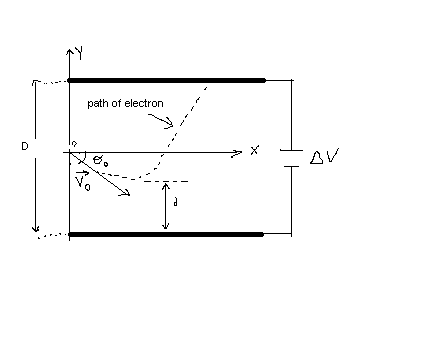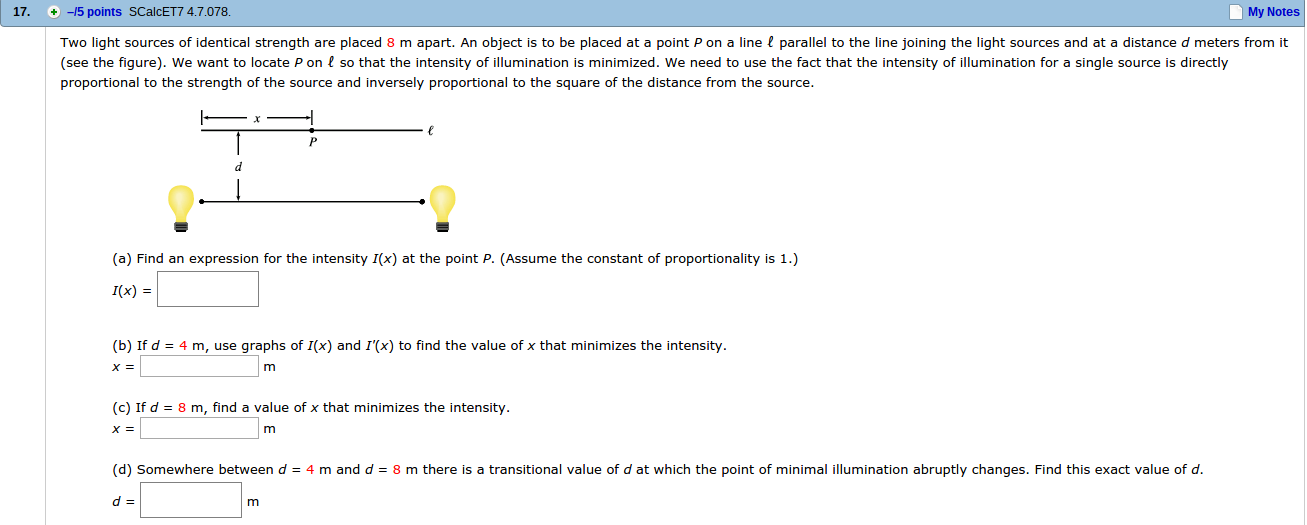Ask question

# Two positive point charges are held in place, 0.74m apart.They are then moved so that their electric potential energydoubles. What is the new sparation between the charges.# Two positive point charges are held in place, 0.74m apart.They are then moved so that their electric potential energydoubles. What is the new sparation between the charges.

Question
Otherasked 2021-03-10
Two positive point charges are held in place, 0.74m apart.They are then moved so that their electric potential energydoubles. What is the new sparation between the charges.

## Answers (1)2021-03-12
if two charges $$\displaystyle+{q}_{{{1}}}\ {\quad\text{and}\quad}\ +{q}_{{{2}}}$$ are seprated by a distance , the potential energy of the systemis
$$\displaystyle{U}={\left(\frac{{1}}{{4}}\pi\epsilon{o}\right)}{\left({q}_{{{1}}}\frac{{q}_{{{2}}}}{{d}}\right)}$$
initiallt d = 0.74m
if U = 2U then the distance between two charges is d/2
i.e., d = 0.37m

### Relevant Questionsasked 2021-01-07
Two positive point charges are held in place, 0.74m apart.They are then moved so that their electric potential energydoubles. What is the new sparation between the charges.asked 2021-05-12
An electron is fired at a speed of $$\displaystyle{v}_{{0}}={5.6}\times{10}^{{6}}$$ m/s and at an angle of $$\displaystyle\theta_{{0}}=–{45}^{\circ}$$ between two parallel conductingplates that are D=2.0 mm apart, as in Figure. Ifthe potential difference between the plates is $$\displaystyle\triangle{V}={100}\ {V}$$, determine (a) how close d the electron will get to the bottom plate and (b) where the electron will strike the top plate.asked 2021-03-29
Two stationary point charges +3 nC and + 2nC are separated bya distance of 50cm. An electron is released from rest at a pointmidway between the two charges and moves along the line connectingthe two charges. What is the speed of the electron when it is 10cmfrom +3nC charge?
Besides the hints I'd like to ask you to give me numericalsolution so I can verify my answer later on. It would be nice ifyou could write it out, but a numerical anser would be fine alongwith the hint how to get there.asked 2021-03-30
A potential difference of 480V is established between largeparallel, metal plates. Let the potential of one plate be 480V andthe other be 0V. The plates are separated by d = 1.70cm.
a) Sketch the equipotential surfaces that correspond to 0, 120,240, 360, and 480 V.
b) In your sketch, show the electric field lines. Does yoursketch confirm that the field lines and surfaces are mutually perpendicular?asked 2021-02-10
Two light sources of identical strength are placed 8 m apart. An object is to be placed at a point P on a line ? parallel to the line joining the light sources and at a distance d meters from it (see the figure). We want to locate P on ? so that the intensity of illumination is minimized. We need to use the fact that the intensity of illumination for a single source is directly proportional to the strength of the source and inversely proportional to the square of the distance from the source.asked 2021-04-25
The unstable nucleus uranium-236 can be regarded as auniformly charged sphere of charge Q=+92e and radius $$\displaystyle{R}={7.4}\times{10}^{{-{15}}}$$ m. In nuclear fission, this can divide into twosmaller nuclei, each of 1/2 the charge and 1/2 the voume of theoriginal uranium-236 nucleus. This is one of the reactionsthat occurred n the nuclear weapon that exploded over Hiroshima, Japan in August 1945.
A. Find the radii of the two "daughter" nuclei of charge+46e.
B. In a simple model for the fission process, immediatelyafter the uranium-236 nucleus has undergone fission the "daughter"nuclei are at rest and just touching. Calculate the kineticenergy that each of the "daughter" nuclei will have when they arevery far apart.
C. In this model the sum of the kinetic energies of the two"daughter" nuclei is the energy released by the fission of oneuranium-236 nucleus. Calculate the energy released by thefission of 10.0 kg of uranium-236. The atomic mass ofuranium-236 is 236 u, where 1 u = 1 atomic mass unit $$\displaystyle={1.66}\times{10}^{{-{27}}}$$ kg. Express your answer both in joules and in kilotonsof TNT (1 kiloton of TNT releases 4.18 x 10^12 J when itexplodes).asked 2020-11-17
Two positive point charges are fixed distance apart. the sum of their charges is QT. What charge must each have in order to (a) maximize the electric force between them, and(b) minimize it?asked 2021-05-18
The student engineer of a campus radio station wishes to verify the effectivencess of the lightning rod on the antenna mast. The unknown resistance $$\displaystyle{R}_{{x}}$$ is between points C and E. Point E is a "true ground", but is inaccessible for direct measurement because the stratum in which it is located is several meters below Earth's surface. Two identical rods are driven into the ground at A and B, introducing an unknown resistance $$\displaystyle{R}_{{y}}$$. The procedure for finding the unknown resistance $$\displaystyle{R}_{{x}}$$ is as follows. Measure resistance $$\displaystyle{R}_{{1}}$$ between points A and B. Then connect A and B with a heavy conducting wire and measure resistance $$\displaystyle{R}_{{2}}$$ between points A and C.Derive a formula for $$\displaystyle{R}_{{x}}$$ in terms of the observable resistances $$\displaystyle{R}_{{1}}$$ and $$\displaystyle{R}_{{2}}$$. A satisfactory ground resistance would be $$\displaystyle{R}_{{x}}{<}{2.0}$$ Ohms. Is the grounding of the station adequate if measurments give $$\displaystyle{R}_{{1}}={13}{O}{h}{m}{s}$$ and R_2=6.0 Ohms?asked 2021-05-02
Suppose electrons enter the uniform electric field midwaybetween two plates, moving at an upward 45 degree angle as shown.What maximum speed can the electrons have if they are to avoidstriking the upper plate. Ignore fringing of the field. Upper plate is negatively charged bottom plate is positivelycharged length of plate is 6cm and distance from top of top plateto bottom of bottom plate is 1cm.
$$\displaystyle{E}={5.0}\times{10}^{{3}}\frac{{N}}{{C}}$$asked 2021-03-23
Five distinct numbers are randomly distributed to players numbered 1 through 5. Whenever two players compare their numbers, the one with the higher one is declared the winner. Initially, players 1 and 2 compare their numbers; the winner then compares with player 3, and so on. Let X denoted the number of times player 1 is a winner. Find P{X = i}, i = 0,1,2,3,4.
...